Home Practice
For learners and parents For teachers and schools
Textbooks
Full catalogue
Pricing SupportLog in

We think you are located in United States. Is this correct?

# 14.5 Probability identities

## 14.5 Probability identities (EMA82)

temp text

By definition, the sample space contains all possible outcomes of an experiment. So we know that the probability of observing an outcome from the sample space is $$\text{1}$$.

$P\left(S\right)=1$

We can calculate the probability of the union of two events using:

$P\left(A\cup B\right) = P\left(A\right) + P\left(B\right) - P\left(A\cap B\right)$

We will prove this identity using the Venn diagrams given above.

For each of the $$\text{4}$$ terms in the union and intersection identity, we can draw the Venn diagram and then add and subtract the different diagrams. The area of a region represents its probability.

We will do this for the first column of the Venn diagram figure given previously. You should also try it for the other columns.

 $$P\left(A\right)$$ $$+$$ $$P\left(B\right)$$ $$-$$ $$P\left(A\cap B\right)$$ $$=$$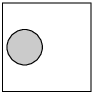$$+$$ $$\big($$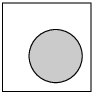$$-$$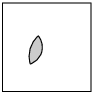$$\big)$$ $$=$$$$+$$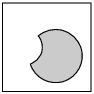$$=$$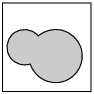$$=$$ $$P\left(A\cup B\right)$$

This video gives an example of how we can add probabilities together.

Video: 2GWN

## Worked example 6: Union and intersection of events

Relate the probabilities of events $$A$$ and $$B$$ from Example 4 (two rolled dice) and show that they satisfy the identity

$P\left(A\cup B\right) = P\left(A\right) + P\left(B\right) - P\left(A\cap B\right)$

### Write down the probabilities of the two events, their union and their intersection

From the Venn diagram in Example 4, we can count the number of outcomes in each event. To get the probability of an event, we divide the size of the event by the size of the sample space, which is $$n\left(S\right)=36$$.

$\begin{array}{rll} P\left(A\right) & = \dfrac{n\left(A\right)}{n\left(S\right)} & = \dfrac{5}{36} \\ P\left(B\right) & = \dfrac{n\left(B\right)}{n\left(S\right)} & = \dfrac{11}{36} \\ P\left(A\cap B\right) & = \dfrac{n\left(A\cap B\right)}{n\left(S\right)} & = \dfrac{2}{36} \\ P\left(A\cup B\right) & = \dfrac{n\left(A\cup B\right)}{n\left(S\right)} & = \dfrac{14}{36} \end{array}$

### Write down and check the identity

\begin{align*} P\left(A\cup B\right) & = P\left(A\right) + P\left(B\right) - P\left(A\cap B\right) \\ \text{RHS } & = \frac{14}{36} \\ \text{LHS } & = \frac{5}{36} + \frac{11}{36} - \frac{2}{36} \\ & = \frac{5}{36} + \frac{9}{36} \\ & = \frac{14}{36} \\ \therefore \text{RHS} & = \text{LHS} \end{align*}
Textbook Exercise 14.5

A group of learners is given the following event sets:

$\begin{array}{|l|c|c|c|c|} \hline \text{Event Set } A & 1 & 2 & 5 & 6 \\ \hline \end{array}$ $\begin{array}{|l|c|} \hline \text{Event Set } B & 3 \\ \hline \end{array}$

$\begin{array}{|l|c|} \hline \text{Event Set } A \cap B & \text{empty} \\ \hline \end{array}$

The sample space can be described as $$\{ n:n \text{ } \epsilon \text{ } \mathbb{Z}, \text{ } 1 \leq n \leq 6 \}$$.

They are asked to calculate the value of $$P(A \cup B)$$. They get stuck, and you offer to calculate it for them. Give your answer as a decimal number, rounded to two decimal places.

$P(A \cup B) = P(A) + P(B) - P(A \cap B)$

Identify variables needed: \begin{align*} P(A) & = \frac{n(A)}{n(S)} = \frac{4}{6} = \text{0,67} \\ P(B) & = \frac{n(B)}{n(S)} = \frac{1}{6} = \text{0,17} \\ P(A \cap B) & = \frac{n(A \cap B)}{n(S)} = \frac{0}{6} = \text{0} \end{align*}

Calculate $$P(A \cup B)$$: \begin{align*} P (A \cup B) & = P(B) + P(A) - P(A \cap B) \\ & = (\text{0,17}) + (\text{0,67}) - (\text{0}) \\ & = \text{0,83} \end{align*}

Therefore, the value of $$P(A \cup B)$$ is $$\text{0,83}$$.

A group of learners is given the following event sets:

$\begin{array}{|l|c|c|c|} \hline \text{Event Set } A & 1 & 2 & 6 \\ \hline \end{array}$ $\begin{array}{|l|c|c|} \hline \text{Event Set } B & 1 & 5 \\ \hline \end{array}$ $\begin{array}{|l|c|c|c|c|} \hline \text{Event Set } A \cup B & 1 & 2 & 5 & 6 \\ \hline \end{array}$

The sample space can be described as $$\{ n:n \text{ } \epsilon \text{ } \mathbb{Z}, \text{ } 1 \leq n \leq 6 \}$$.

They are asked to calculate the value of $$P(A \cap B)$$. They get stuck, and you offer to calculate it for them. Give your answer as a decimal number, rounded to two decimal value.

$P(A \cup B) = P(A) + P(B) - P(A \cup B)$

Make $$P(A \cap B)$$ the subject, and we get: \begin{align*} P (A \cap B) & = P(B) + P(A) - P(A \cup B) \end{align*}

Identify variables needed: \begin{align*} P(A) & = \frac{n(A)}{n(S)} = \frac{3}{6} = \text{0,5} \\ P(B) & = \frac{n(B)}{n(S)} = \frac{2}{6} = \text{0,33} \\ P(A \cup B) & = \frac{n(A \cup B)}{n(S)} = \frac{4}{6} = \text{0,67} \end{align*}

Calculate $$P(A \cap B)$$: \begin{align*} P (A \cap B) & = P(B) + P(A) - P(A \cup B) \\ & = (\text{0,33}) + (\text{0,5}) - (\text{0,67}) \\ & = \text{0,17} \end{align*}

Therefore, the value of $$P(A \cap B)$$ is $$\text{0,17}$$.